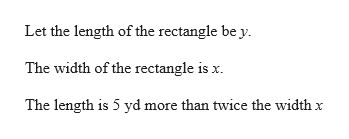# The length of a rectangle is 5 yd more than twice the width x. The area is 738 yd2.Write an equation in terms of x that represents the given relationship

Question
39 views

The length of a rectangle is 5 yd more than twice the width x. The area is 738 yd2.

Write an equation in terms of x that represents the given relationship

check_circle

Step 1help_outlineImage TranscriptioncloseLet the length of the rectangle be y The width of the rectangle is x. The length is 5 yd more than twice the width x fullscreen

### Want to see the full answer?

See Solution

#### Want to see this answer and more?

Solutions are written by subject experts who are available 24/7. Questions are typically answered within 1 hour.*

See Solution
*Response times may vary by subject and question.
Tagged in
MathAlgebra

### Other